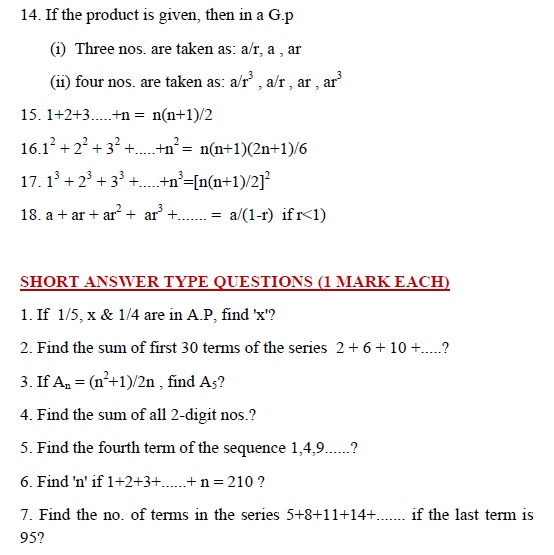# SCC Education

## concept of Sequence and series with important questions

Sequence and seriesSeries-formulas

The-binomial-theorem

solved-problems-on-limits-and-continuity

geometric-progressions

Basic-point-formulas-distance-midpoint

Two-point-form-normal-form parametric

limit-questions

set-relation-function-part1

permutation-combination

straight-lines

Three-diamensional-geometry

Trignometry

hyperbola